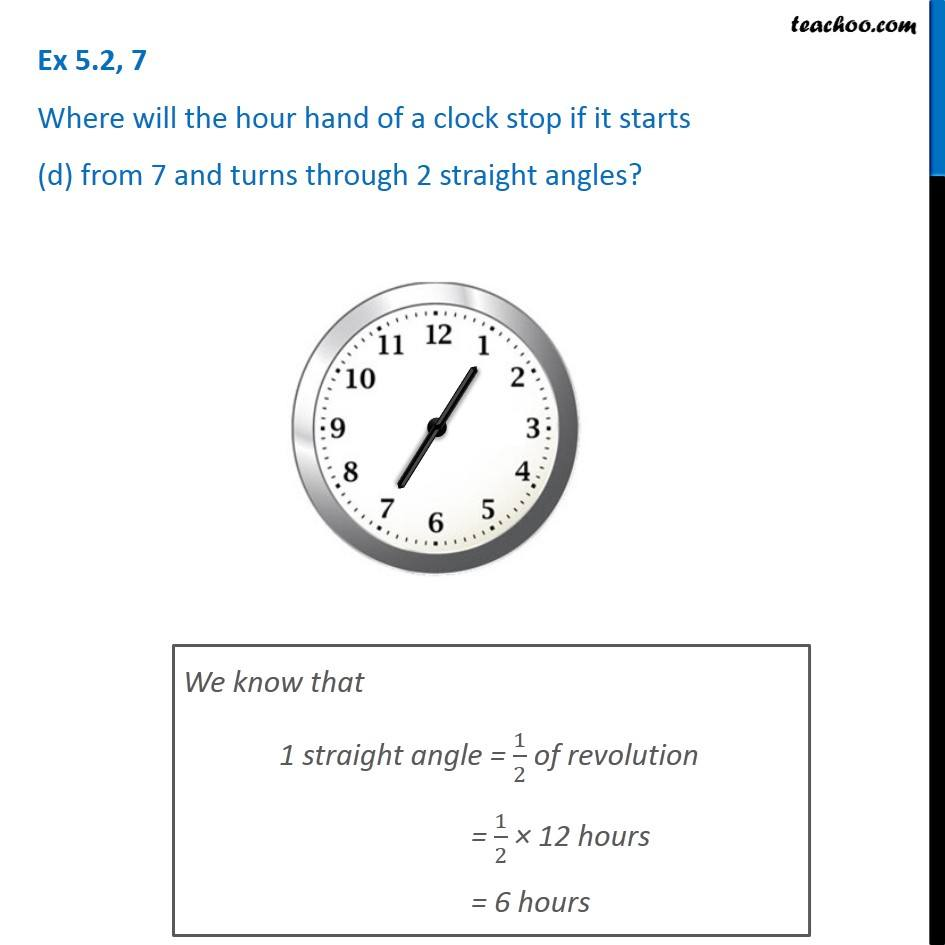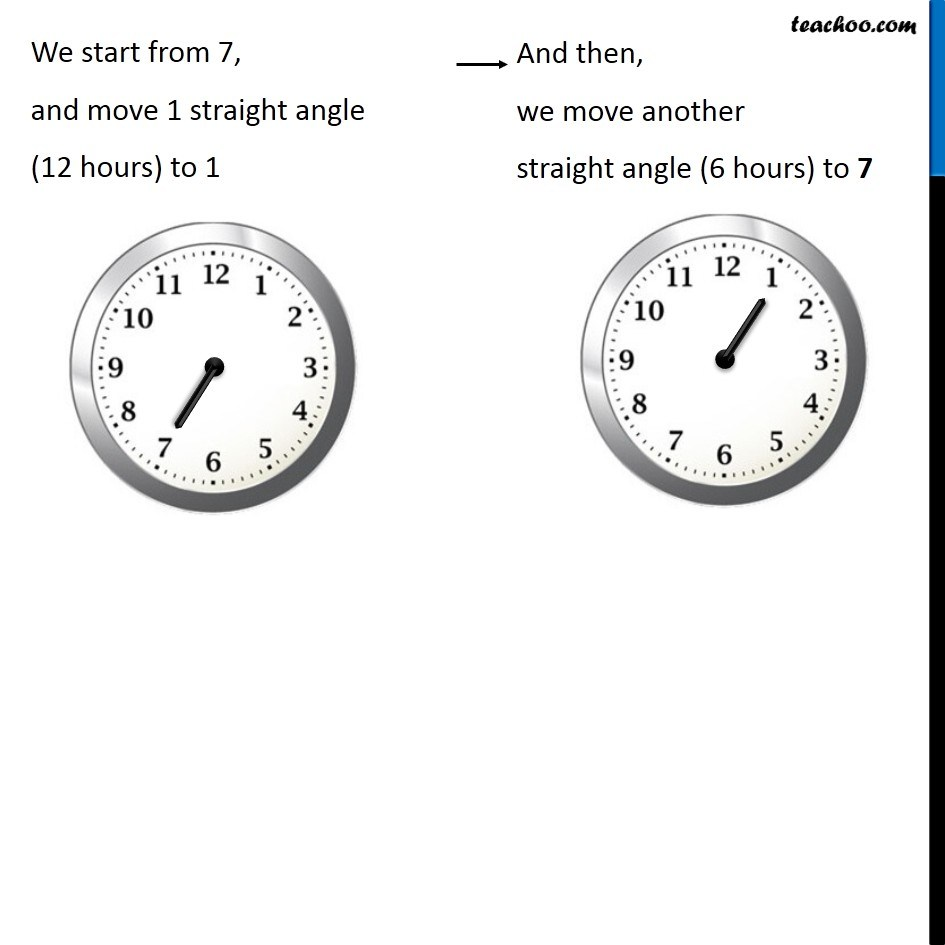1. Chapter 5 Class 6 Understanding Elementary Shapes
2. Serial order wise
3. Ex 5.2

Transcript

Ex 5.2, 7 Where will the hour hand of a clock stop if it starts (d) from 7 and turns through 2 straight angles? We know that 1 straight angle = 1/2 of revolution = 1/2 × 12 hours = 6 hours We start from 7, and move 1 straight angle (12 hours) to 1 We start from 7, and move 1 straight angle (12 hours) to 1